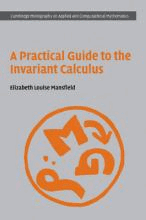# A Practical Guide to the Invariant Calculus by Mansfield, Elizabeth Louise

• R 450.00
Unit price per
Tax included.

ISBN: 9780521857017

CAMBRIDGE UNIVERSITY PRESS, 30 June 2010

Hardback, 260 pages

This book explains recent results in the theory of moving frames that concern the symbolic manipulation of invariants of Lie group actions. In particular, theorems concerning the calculation of generators of algebras of differential invariants, and the relations they satisfy, are discussed in detail. The author demonstrates how new ideas lead to significant progress in two main applications: the solution of invariant ordinary differential equations and the structure of Euler-Lagrange equations and conservation laws of variational problems. The expository language used here is primarily that of undergraduate calculus rather than differential geometry, making the topic more accessible to a student audience. More sophisticated ideas from differential topology and Lie theory are explained from scratch using illustrative examples and exercises. This book is ideal for graduate students and researchers working in differential equations, symbolic computation, applications of Lie groups and, to a lesser extent, differential geometry.